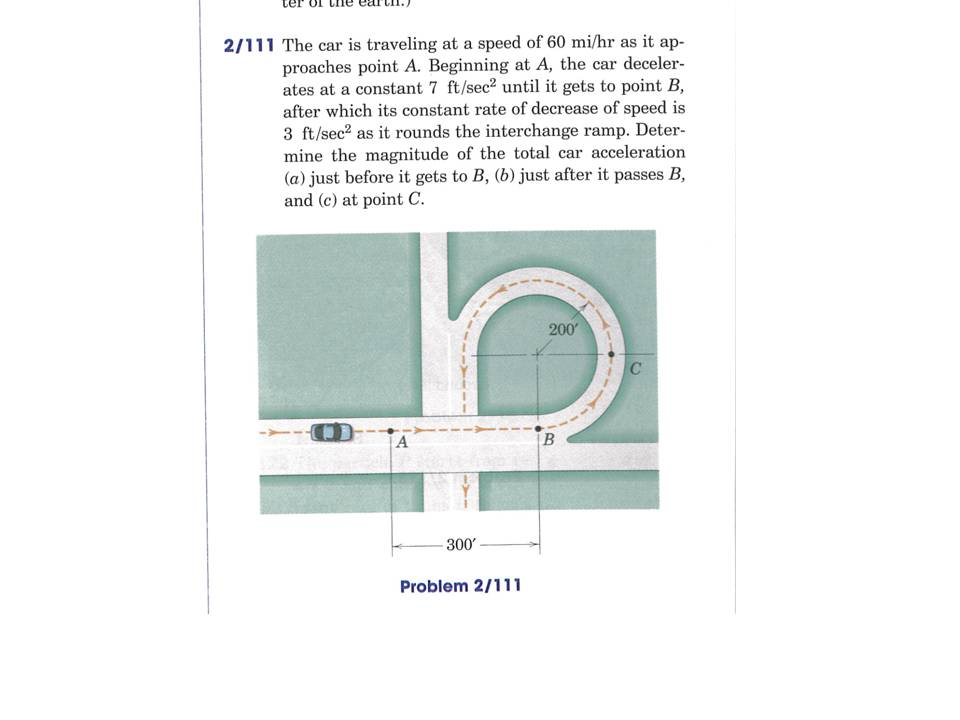# Question The car is traveling at a speed of 60 mi/hr as it approaches point A. Beginning at A, the car decelerates at a constant 7ft/sec^2 until it gets to point B, after which its constant rate of decrease of speed is 3 ft/sec^2 as it rounds the interchange ramp. Determine the magnitue of the total car acceleration a) just before it gets to B, b) just after it passes B, and c) at point C.CLWOMY The Asker · Mechanical EngineeringThe car is traveling at a speed of 60 mi/hr as it approaches point A. Beginning at A, the car decelerates at a constant 7ft/sec^2 until it gets to point B, after which its constant rate of decrease of speed is 3 ft/sec^2 as it rounds the interchange ramp. Determine the magnitue of the total car acceleration a) just before it gets to B, b) just after it passes B, and c) at point C.

Transcribed Image Text: Ee 2/111 The car is traveling at a speed of 60 mi/hr as it ap- proaches point A. Beginning at A, the car deceler- ates at a constant 7 ft/sec? until it gets to point B, after which its constant rate of decrease of speed is 3 ft/sec? as it rounds the interchange ramp. Deter- mine the magnitude of the total car acceleration (a) just before it gets to B, (b) just after it passes B, and (c) at point C. Problem 2/111
More
Transcribed Image Text: Ee 2/111 The car is traveling at a speed of 60 mi/hr as it ap- proaches point A. Beginning at A, the car deceler- ates at a constant 7 ft/sec? until it gets to point B, after which its constant rate of decrease of speed is 3 ft/sec? as it rounds the interchange ramp. Deter- mine the magnitude of the total car acceleration (a) just before it gets to B, (b) just after it passes B, and (c) at point C. Problem 2/111